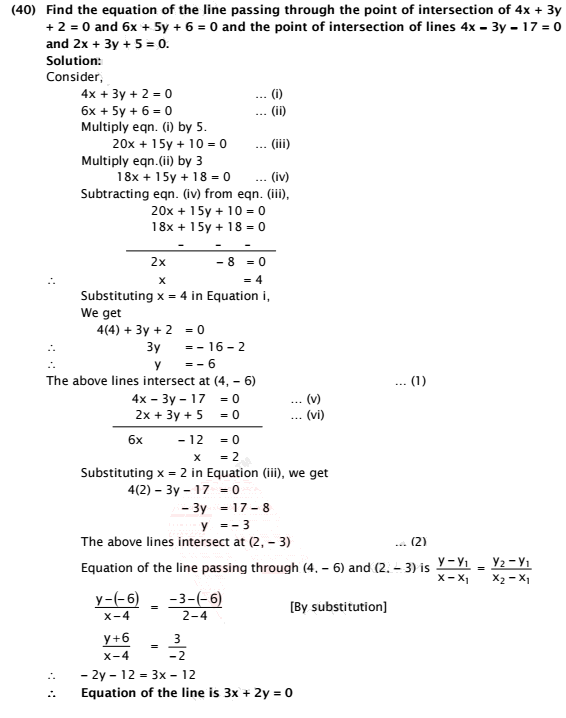### Find the equation of the line passing through the point of intersection of 4x + 3y + 2 = 0 and 6x + 5y + 6 = 0 and the point of intersection of lines 4x − 3y − 17 = 0 and 2x + 3y + 5 = 0.